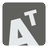Skew-symmetric Matrix
Elementary
English DE HU JA RU

In mathematics, particularly in linear algebra, a skew-symmetric matrix (also known as an antisymmetric or antimetric) is a square matrix A which is transposed and negative. This means that it satisfies the equation A = −AT. If the entry in the i-th row and j-th column is aij, i.e. A = (aij) then the symmetric condition becomes aij = −aji.

You should determine whether the specified square matrix is skew-symmetric or not.

You can find more details on Skew-symmetric matrices on its Wikipedia page.

Input: A square matrix as a list of lists with integers.

Output: If the matrix is skew-symmetric or not as a boolean.

Example:

```checkio([
[ 0,  1,  2],
[-1,  0,  1],
[-2, -1,  0]]) == True
checkio([
[ 0,  1, 2],
[-1,  1, 1],
[-2, -1, 0]]) == False
checkio([
[ 0,  1, 2],
[-1,  0, 1],
[-3, -1, 0]]) == False
```

How it is used: Skew-symmetric matrices can be useful for the cross product, mathematical operations which are used for the calculation of force movements. Matrixes are the basis of linear algebra and vector graphics.

Precondition: 0 < N < 5